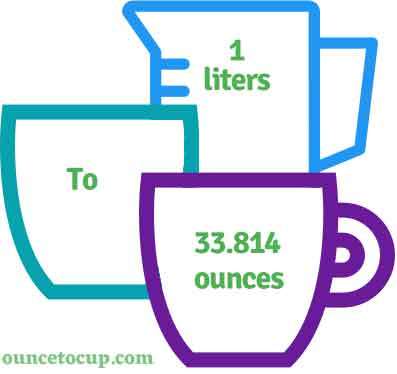# Liters to Ounces (l to oz conversion)

Are you cooking your favorite dish? The detailed chart in the recipe includes the calculation of the 1 liter to ounce conversion.  Don't worry; use this calculator to determine how many 1 liter equals ounces in a minute.  This 1 l to oz converter gives an exact measurement for any recipe you prepare.

Liter Value:

l

Ounce Value:

oz

1 Liter = 33.814 Ounce
(1 l = 33.814 oz)

Try our auto 1 Liter to Ounce Calculator (Without Convert Button), Just change the first field value and you got final value.## How many oz is a l?

We know that the mass value of 1 l is equal to 33.814 oz. If you want to convert 1 fluid l to an equal number of oz, just multiply the mass value by 33.814. Hence, 1 liter is equal to 33.814 oz.

The Answer is: 1 US Liters = 33.814 US Ounce

1 l = 33.814 oz

Many of them try to search or find an answer for what is 1 liters in oz? So, we’ll start with 1 l to oz conversion to know how big is 1 l.

## How To Calculate 1 liter to oz?

To calculate 1 liters to an equal number of ounce, simply follow the steps below.

Liters to Fluid Ounces formula is:

Ounce = Liter * 33.814

Assume that we are finding out how many oz were found in 1 fl l of water, multiply by 33.814 to get the result.

Applying to Formula: oz = 1 l * 33.814 = 33.814 oz.

## How To Convert l to oz?

• To convert liters to oz,
• Simply multiply the liter value by 33.814
• Applying to the formula, oz = 1 liters * 33.814 [1x33.814].
• Hence, 1 liters is equal to 33.814 oz.

## Some quick table references for liter to ounce conversions:

Liter [l]Ounce [oz]
1 l33.814 oz
2 l67.628 oz
3 l101.442 oz
4 l135.256 oz
5 l169.07 oz
6 l202.884 oz
7 l236.698 oz
8 l270.512 oz
9 l304.326 oz
10 l338.14 oz
11 l371.954 oz
12 l405.768 oz
13 l439.582 oz
14 l473.396 oz
15 l507.21 oz

## Reverse Calculation: How many liters are in a oz?

• To convert 1 oz to l,
• Simply divide the 1 oz by 33.814.
• Then, applying the formula, l = 1 oz / 33.814 [1/33.814 = 0.0295735].
• Hence, 1 ounce is equal to 0.0295735 liter.

### Related Converter:

Formula: liter to oz

oz = liter * 33.814

Applying to Formula,

oz = 1*33.814 = 33.814

1 l = 33.814 oz